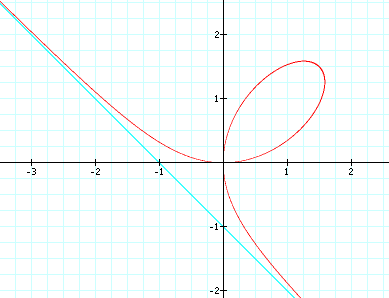# Folium of Descartes

## DescriptionFolium of Descartes is the curve x^3 + y^3 == 3x*y.

## History

This curve is first discussed by Rene Descartes in 1638.

## Formula

Cartesian: `x^3 + y^3 == 3x*y`.

Parametric: `{3*t, 3t^2}/(1 + t^3)`. In this formula, the curve tends to the Origin as t→±∞. The curve tends to ∞ when t→-1.

Polar: `r==(3*Sin[θ]*Cos[θ])/(Sin[θ]^3+Cos[θ]^3)`.

Its asymptote is y==x-1.

## Related Web Sites

Robert Yates: Curves and Their Properties .